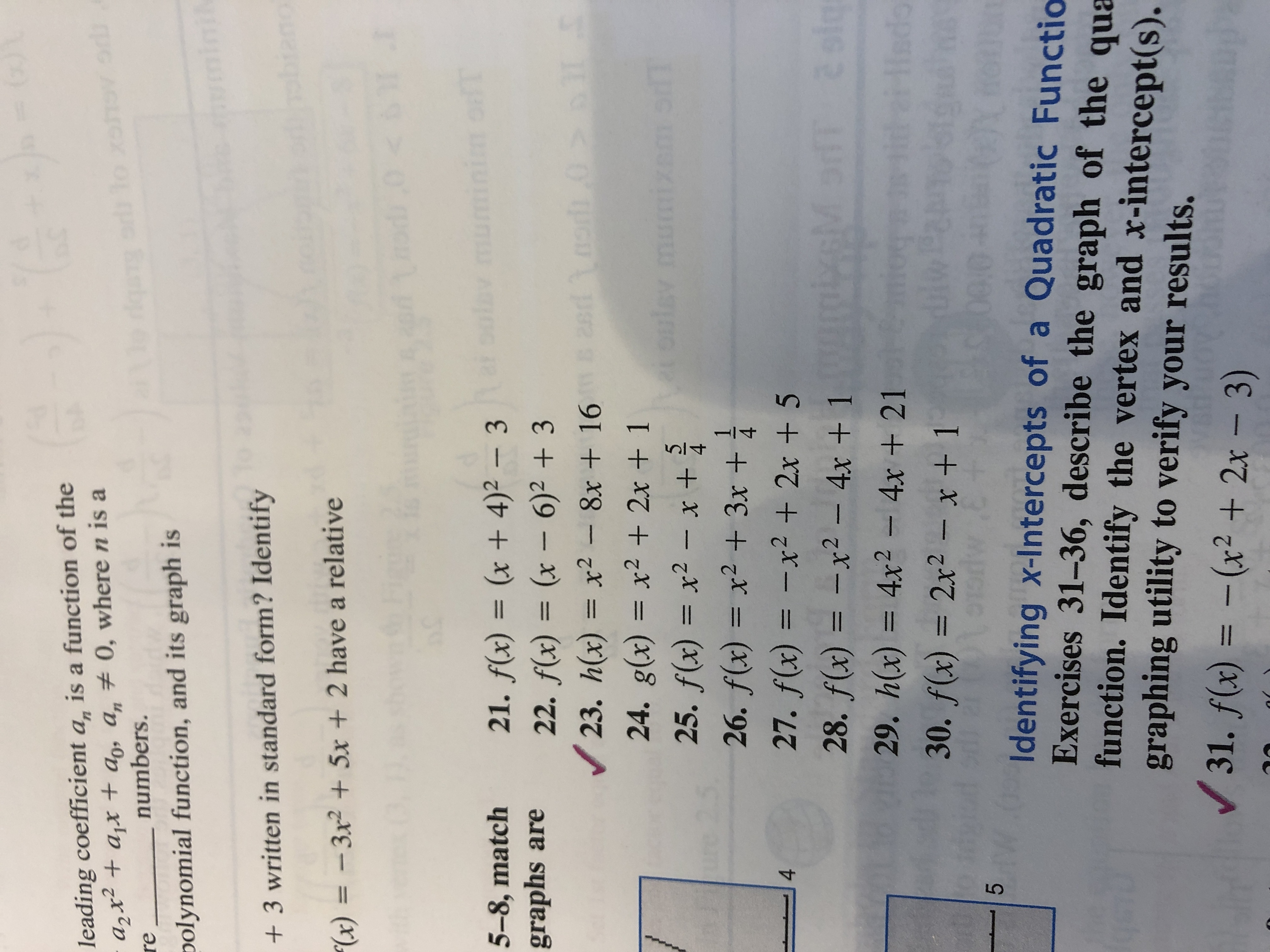# ()1leading coefficient a, is a function of thea2x+ajx t+ a, a, 0, where n is areqao To xeh o dnumbers.polynomial function, and its graph is3 written in standard form? Identify"(x) = -3x2 + 5x + 2 have a relative

Question
1 views

Number 29, identify the vertexhelp_outlineImage Transcriptionclose()1 leading coefficient a, is a function of the a2x+ajx t+ a, a, 0, where n is a re qao To xeh o d numbers. polynomial function, and its graph is 3 written in standard form? Identify "(x) = -3x2 + 5x + 2 have a relative
check_circle

Step 1

Given,

Step 2

Now,

Step 3

Therefore x-coordinate o...

### Want to see the full answer?

See Solution

#### Want to see this answer and more?

Solutions are written by subject experts who are available 24/7. Questions are typically answered within 1 hour.*

See Solution
*Response times may vary by subject and question.
Tagged in

### Other